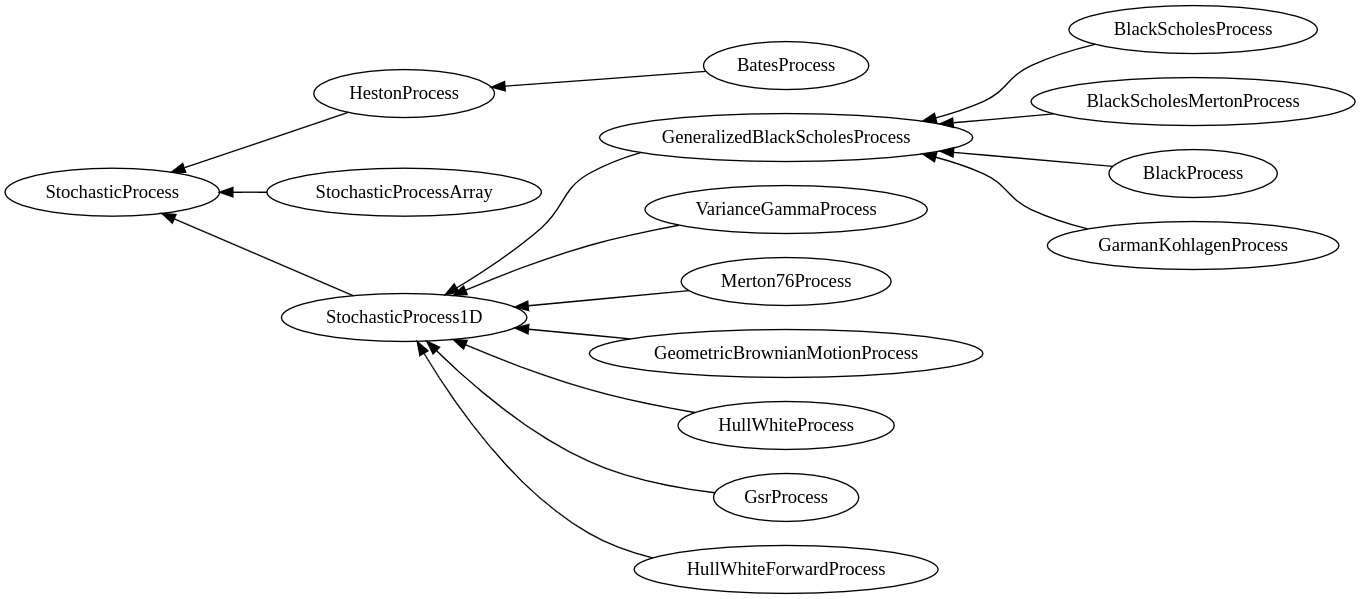# QuantLib 金融计算——随机过程之概述

import QuantLib as ql

print(ql.__version__)

1.12


## 框架C++ 版本的实现提供了更多具体的随机过程。

• HestonProcess：特殊的二维随机过程——Heston 过程；
• BatesProcess：一种带跳跃的 Heston 过程；
• StochasticProcessArray：描述一般的多维随机过程；
• StochasticProcess1D：描述常用的若干一维随机过程。
• GeneralizedBlackScholesProcess：Black-Scholes 框架下四种最常用的随机过程
• BlackScholesProcess$$d \ln S ( t ) = \left( r ( t ) - \frac { \sigma ( t , S ) ^ { 2 } } { 2 } \right) d t + \sigma d W _ { t }$$
• BlackScholesMertonProcess$$d \ln S ( t , S ) = \left( r ( t ) - q ( t ) - \frac { \sigma ( t , S ) ^ { 2 } } { 2 } \right) d t + \sigma d W _ { t }$$
• BlackProcess$$d \ln S ( t ) = - \frac { \sigma ( t , S ) ^ { 2 } } { 2 } d t + \sigma d W _ { t }$$
• GarmanKohlagenProcess$$d \ln S ( t ) = \left( r ( t ) - r _ { f } ( t ) - \frac { \sigma ( t , S ) ^ { 2 } } { 2 } \right) d t + \sigma d W _ { t }$$
• VarianceGammaProcess
• Merton76Process
• GeometricBrownianMotionProcess$$d S ( t , S ) = \mu S d t + \sigma S d W _ { t }$$
• HullWhiteProcess
• HullWhiteForwardProcess
• GsrProcess

$d \mathrm S_t = \mu \left( t , S_t \right) \mathrm d t + \sigma \left( t , \mathrm S_t \right) d \mathrm { W }_t$

quantlib-python 默认的离散化方法是 Euler 方法：

$S \left( t + \Delta t \right) = \mu \left( t , S_t \right) \Delta t + \sigma \left( t , S_t \right) \Delta W_t$

## 用法与接口

StochasticProcess 提供下列成员函数：

• size()：整数，返回随机过程的维度；
• initialValues()Array，返回数组 $$S_0$$
• drift(t, x)Array，返回数组 $$\mu(t,S_t)$$tx 分别是浮点数和 Array
• diffusion(t, x)Array，返回数组 $$\sigma(t,S_t)$$tx 分别是浮点数和 Array
• expectation(t0, x0, dt)Array，根据具体的离散方法返回数组 $$E \left( S_{ t_0 + \Delta t} | S_{ t_0 } = x_0 \right)$$t0dt 是浮点数，x0Array
• stdDeviation(t0, x0, dt)Matrix，根据具体的离散方法返回标准差矩阵 $$Std \left( S_{ t_0 + \Delta t} | S_{ t_0 } = x_0 \right)$$t0dt 是浮点数，x0Array
• covariance(t0, x0, dt)Matrix，根据具体的离散方法返回协方差矩阵 $$Cov \left( S_{ t_0 + \Delta t} | S_{ t_0 } = x_0 \right)$$t0dt 是浮点数，x0Array
• evolve(t0, x0, dt, dw)Array，根据 $$S_{ t_0}$$ 和 Brownian 运动增量 $$\Delta W$$ 产生 $$S_{ t_0 + \Delta t}$$，默认返回 $$E \left( \mathrm S_{ t_0 + \Delta t } | S_{ t_0 } \right) + \sigma \left( \mathrm S_{ t_0 + \Delta t } | S_{ t_0 } \right) \Delta \mathrm { W }$$，其中 $$\sigma$$ 是标准差（矩阵）.

## 扩展阅读

《QuantLib 金融计算》系列合集

posted @ 2019-01-22 23:29  xuruilong100  阅读(1387)  评论(0编辑  收藏  举报